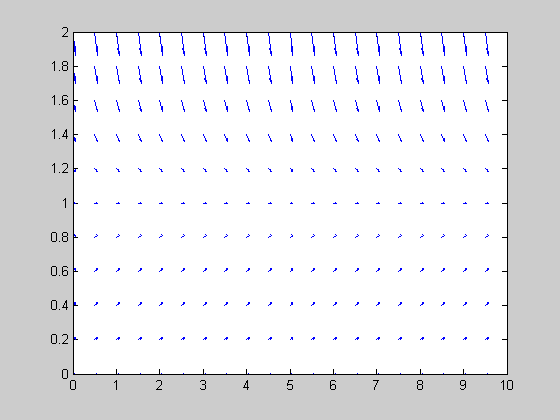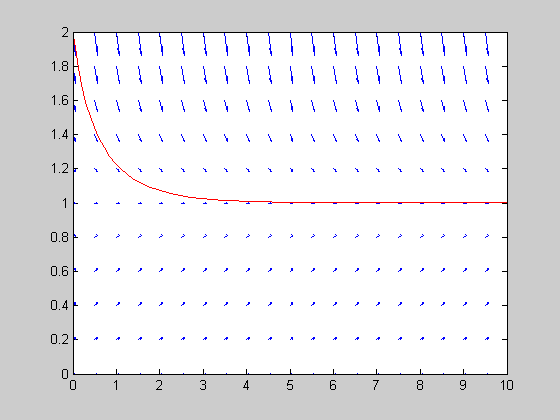# Calculate and plot slope field dx/dt of a 1D system "integrate1D"

## Contents

close figures and clears the memory

```close all, clear
```

## Definition of the variables range t, x and mesh grid to points (tx, xx) to calculate the slope field

```%upper integration time limit
tk=10;
t = 0:.5:tk;
x = 0:.2:2;
% Definition of the rectangular mesh grid for t, x
[tx,xx] = meshgrid(t,x);
```

## Calculation of the slope field

Awkward definition of dt used in calculation of dx

```dt=1;
% calculation of the dx/dt given by the equation
dxdt=xx.*(1-xx);
% calculation of coresponding dx and dt
dx=dxdt*dt;
td=ones(length(x),length(t)) * dt;
```

## Plot the slope field (dt,dx) in the points tx, xx

```quiver(tx,xx,td,dx,0.3);
% fixing the figure
axis([0 tk 0 2]);
hold;
```
```Current plot held
```## Integrate and plot the trajectory into the slope field t=0, x=2

initial value t=0, x=2

```[t,y]=ode45(@integrate1D,[0 tk],);
plot(t,y(:,1),'r');
```## Integrate and plot the trajectory into the slope field t=0, x=0.01

```%initial value t=0, x=0.1
[t,y]=ode45(@integrate1D,[0 tk],[0.01]);
plot(t,y(:,1),'r');
```## plot the trajectory into the slope field t=2, x=2

```%initial value t=2, x=2
[t,y]=ode45(@integrate1D,[0 tk],);
plot(t+2,y(:,1),'r');
```